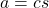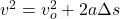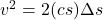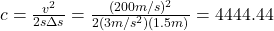## If the acceleration of the projective is: a = c s m/s 2 Where c is a constant that depends on the initial gas pressure behind the projectile

Question

If the acceleration of the projective is: a = c s m/s 2 Where c is a constant that depends on the initial gas pressure behind the projectile. The initial position of the projectile is s=1.5m and the projectile is initially at rest. The projectile accelerates until it reaches the end of the barrel at s=3m. What is the value of the constant c such that the projectile leaves the barrel with a velocity of 200 m/s?

in progress 0
5 months 2021-09-05T12:17:35+00:00 1 Answers 0 views 0

## Answers ( )

c = 4,444.44

Explanation:

You have the following expression for the acceleration of the projectile:(1)

s: distance to the ground of the projectile

To find the value of the constant c you use the following formula:(2)

vo: initial  velocity = 0 m/s

v: final speed = 200 m/s

Δs: distance traveled by the projectile = 3m – 1.5m = 1.5m

You replace the expression (1) into the expression (2):You do the constant c in the last equation, then you replace the values of v, s and Δs: